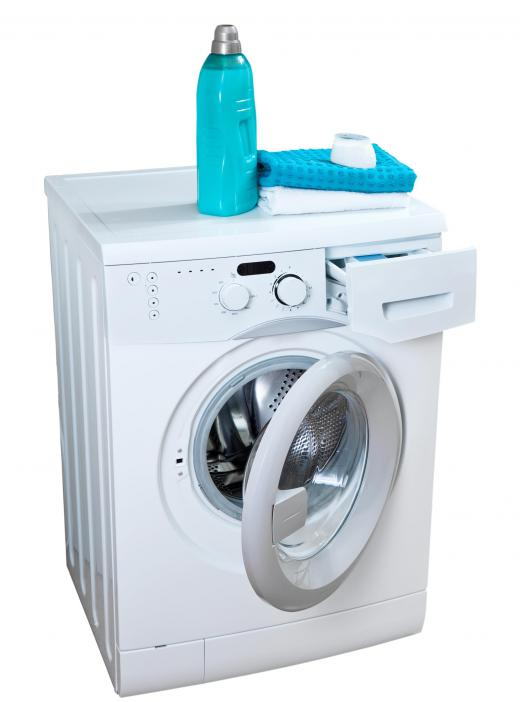# How Do I Measure Washing Machine Dimensions?

Anna B. Smith
Anna B. SmithIn a top loading washing machine, the radius is the distance from the inside edge of the tub wall to the center of the agitator cylinder.

To measure washing machine dimensions, the owner can multiply the area of the interior washing machine tub by its height. The dimensions indicate the volume of water each machine can hold. This number is usually measured in cubic feet. To determine the capacity at which one particular machine operates, the owner will need to measure the height and width of his washer's tub. This equation may be used to find the volume of a dryer, as well as the washer.The radius of a front-loading machine is determined by measuring from one top wall to the bottom to locate the diameter, then dividing by half.

To calculate the area of the tub, the owner can use the same mathematical equation that is used to determine the area of a circle. The area of a circle may be found by multiplying pi by the radius of the circle, squared. The radius of a top loading washing machine tub is measured from the inside edge of the tub wall to the center of the agitator cylinder. The radius of a front loading machine may be calculated by measuring from one top wall to the bottom to locate the diameter. This number, when divided in half, yields the radius. To square this number, it is multiplied by itself.

A washer with an interior tub that is 15 inches (38.1 centimeters) deep and has a diameter of 20 inches (50.8 centimeters) will have a radius of 10 inches (25.4 centimeters). The radius of that machine when squared is 100 inches. This number is then multiplied by pi, which is 3.14, to determine the area. 100 x 3.14 = 314 inches. The area of this example washer unit is 314 inches (797.56 centimeters), which can then be used to determine the washing machine dimensions in terms of volume.The dimensions of a washing machine will determine the amount of detergent necessary.

The volume of the machine may next be found by multiplying the height by the area of the interior tub. The area of the example washer was calculated to be 314 inches, which provides a volume of 4,710 cubic inches (11,963.4 centimeters). 314 inches of area x 15 inches in height = 4,710 cubic inches.

The volume of the machine, once calculated in cubic inches, must be converted to cubic feet to find the exact washing machine dimensions. There are 1,728 cubic inches to every cubic foot. The example washer has a volume of 4,710 cubic inches, which can be divided into 2.73 cubic feet. 4,710 cubic inches / 1,728 cubic inches = 2.73 cubic feet (0.077304 cubic meters).

The washing machine dimensions may not always be listed in cubic feet. In such instances, the manufacturer typically provides the machine's specifications in terms of height, width, and depth. Some machines may also have a value for the maximum weight capacity they can hold, often listed in kilograms. This is a measurement of the unit's weight bearing capabilities, and cannot be determined by calculating the interior dimensions, which are a measure of volume.Talentryto

If you have a small laundry room, it is very important to get the exterior dimensions of a washing machine and dryer accurate. Otherwise, you may find yourself returning them for a smaller set that will fit in your area. This is a common mistake made by new homeowners, because it is easy to misjudge small spaces and the large appliances that will go into them. I did this with a refrigerator once, and had to cut out part of a wall to make it fit!

Heavanet

Usually you can find these dimensions in the washing machine owner's manual. However, if you have lost it or you purchase a used washing machine that doesn't have one, the information in this article on doing the measurements yourself are very helpful.